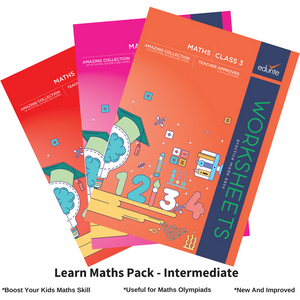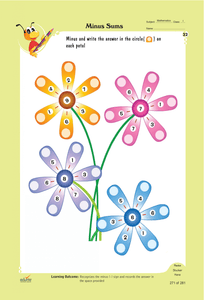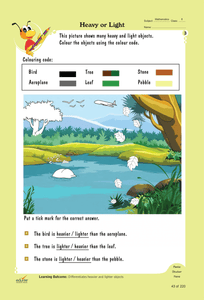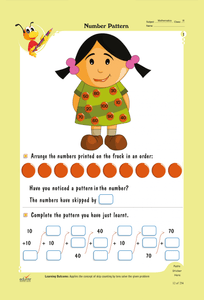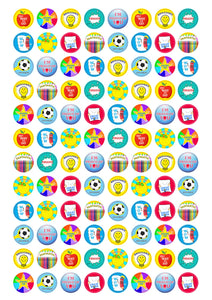# Edurite Learn Maths Pack for Kids Intermediate (3 Book Combo, Ideal for Age 5- 8)

Regular price
Rs. 3,499.00
Sale price
Rs. 2,699.00

Units Sold: 2135

Free Delivery.
Makes Learning Math Fun Again
Successfully Deployed in schools helping more than 4500 children
Rave Reviews from Principals of Schools
Cash on Delivery Available with 7 Day Return Policy

Key features of our worksheets:

• Bright and colorful pages that intrigue students
• Useful for Olympiads and other competitive exams.
• Ideal for Kids who is in class 1 to 3
• Variety of interactive activities that reiterate each concept
• Provide a thorough understanding of the question pattern
• Hard and user-friendly A4 Size pages
• Prepared by the experienced academicians in tune with the updated NCERT syllabus
• FREE Study Plan

Topics covered in Book 1

• Interpretation of given data in the form of an equation
• Practice addition and subtraction of numbers
• Translate visual data into a bar graph and interpret visual data
• Understanding sequencing of numbers
• Comprehend the sequence of activities
• Comprehend the size of objects
• Comprehend the concept of zero
• Deduce that certain objects have fixed numbers
• Comprehend the concept of date by writing the date which comes after a given date
• Compare and recognize the smaller and bigger groups from a given set of objects
• Recognize long-short, thick-thin and heavy-light objects
• Identifying currency, addition, and subtraction
• Differentiate between smaller and larger numbers
• Writing number names
• Practice how to read numbers on the Abacus
• Solving statement problems
• Telling time

Topics covered in Book 2

• Practice addition concepts
• Solve the given word problems
• Practice addition of numbers by regrouping them
• Practice horizontal and vertical addition of three-digit numbers
• Interpret the given visual data and answer the questions
• Identify odd and even numbers
• Practice vertical and horizontal addition of three-digit numbers
• Interpret the given visual data and answer questions
• Demonstrate the understanding of the concept of counting in tens
• Identify odd and even numbers on a number line
• Solve the given problems by using the concept of addition in terms of tens and ones
• Demonstrate the concept of counting in tens and fives
• Count numbers in tens from 10 to 500
• Observe the relationship between time and length
• Identify different kinds of lines
• Identify the kind of lines used to write letters
• Recognize standing and sleeping lines to write numbers
• Create patterns using standing and sleeping lines
• Appreciate the concept of leap year and complete the calendar
• Recognize the ordinal number
• Calculate the total number of objects in terms of tens and ones
• Learning to use a calendar
• Shapes, Cylinders and spheres
• Weight-Heavier and Lighter

Topics covered in Book 3

• Analyze and compare the weight of given objects
• Comprehend the concept of addition and subtraction of small numbers
• Recall the concept of place value and skip counting
• Associate the given number with 10 and solve the given problem
• Add two digit numbers by regrouping them
• Comprehend the commutative property of addition
• Comprehend the given word problem and solve them
• Solve the given addition problems in a given time
• Practice addition of three-digit numbers
• Comprehend and solve problems involving large numbers
• Apply the concept of addition and subtraction to solve the given problem
• Solve the problem mentally using the given information
• Apply the logical reasoning skill to solve the given problem
• Interpret the given data and apply mathematical skills to solve the problem
• Practices skip counting backwards and forwards by tens and fifties
• Observe the given calendar and also comprehend the concept of days and weeks
• Observe the given denomination of money and count them
• Comprehend the concept of billing and apply basic math skills to solve given problems
• Recognize the order of the number pattern given
• Draw and Divide
• Count and divide the objects in groups of 2, 3, 4, and 5
• Writing and Interpreting Division Statements, Multiplication Sentences
• Comprehend the concept of distance and metric system
• Telling time and calculating necessary weight to balance a scale

## Customer Reviews

Based on 5 reviews